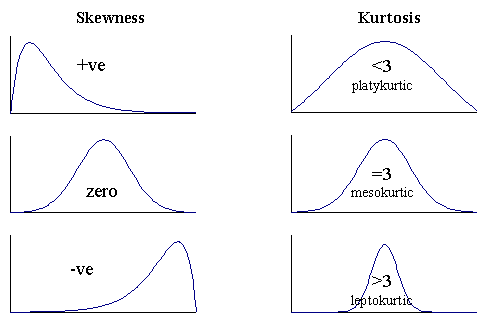Search
•SoniaSamipillai

# Descriptive Statistics - Measures of Shape

Updated: Jul 17, 2021Not everything that can be counted counts, and not everything that counts can be counted.

The moments of a function are quantitative measures related to the shape of the function's graph. If the function is a probability distribution, then the zeroth moment is the total probability (i.e. one), the first moment is the expected value or Mean, the second central moment is the Variance, the third standardized moment is the Skewness, and the fourth standardized moment is the Kurtosis.

The shape of a distribution can be easily observed through Histograms and Density plots. Two important Measures of Shape are:

1. Skewness

2. Kurtosis

Skewness

The formula to compute Skewness is given byUsually just by observing the shape of the histogram of the data, one would be able to see if the data is skewed and if skewed, Is it positively skewed or negatively skewed?. For data skewed to the left, the skewness measure is negative and for the data skewed to the right, the skewness measure is positive. The skewness is zero when the data is symmetric.

In cases where data is skewed, Median is a preferred measure of location rather than mean because mean gets affected by the presence of outliers that brings about skewness in the distribution.Kurtosis

The outliers in a sample, have even more effect on the kurtosis than they do on the skewness and in a symmetric distribution, both tails increase the kurtosis, unlike skewness where they offset each other. There are three types of data shapes; Platykurtic, Mesokurtic, and Leptokurtic. Kurtosis has no units and it is just a number.

1. A normal distribution has kurtosis exactly 3 (excess kurtosis exactly 0). Any distribution with kurtosis approximately 3 is called mesokurtic.

2. A distribution with kurtosis <3 is called platykurtic. Compared to a normal distribution, its tails are shorter and thinner, and often its central peak is lower and broader.

3. A distribution with kurtosis >3 is called leptokurtic. Compared to a normal distribution, its tails are longer and fatter, and often its central peak is higher and sharper.

The shape of the distribution can assist with identifying other descriptive statistics, such as which measure of central tendency is appropriate to use. If the data are normally distributed, the mean, median, and mode are all equal and therefore are all appropriate measures of central tendency. If data are skewed, the median may be a more appropriate measure of central tendency.

Hope this post was helpful!!. If you’re interested to read more, you can subscribe and be notified when the next article is published.